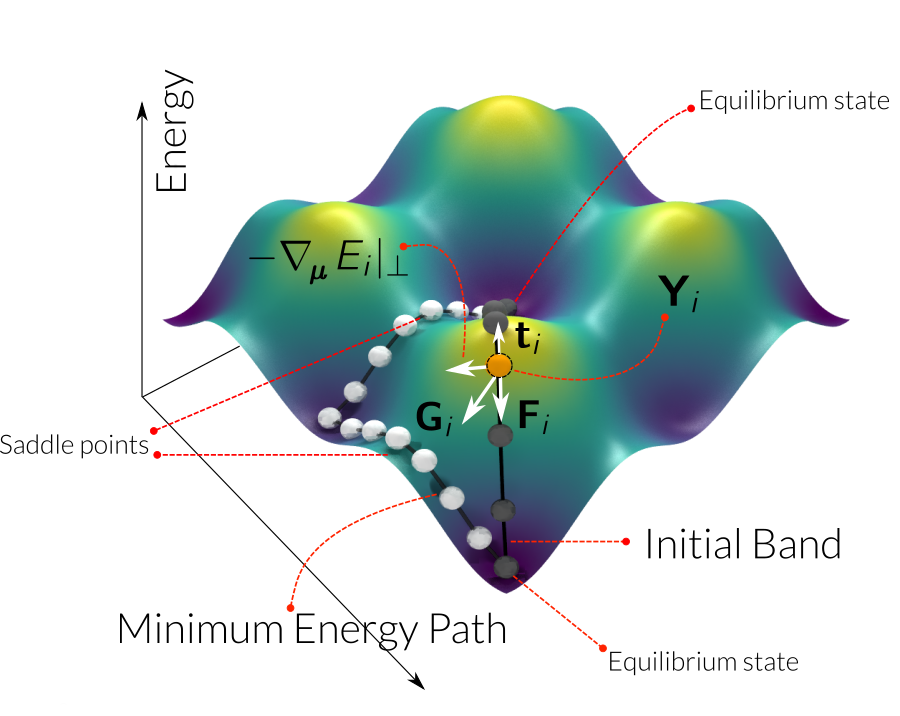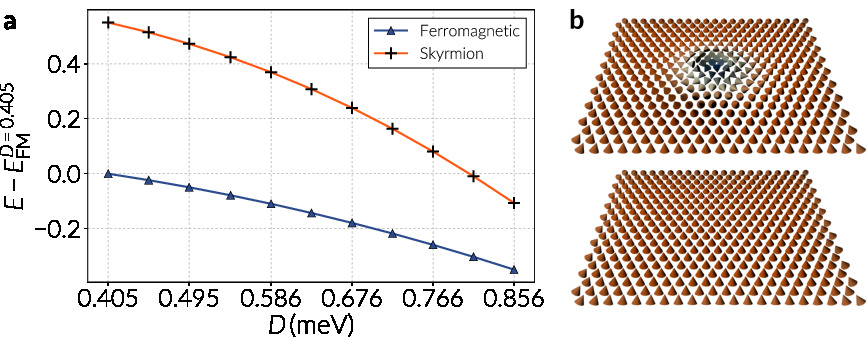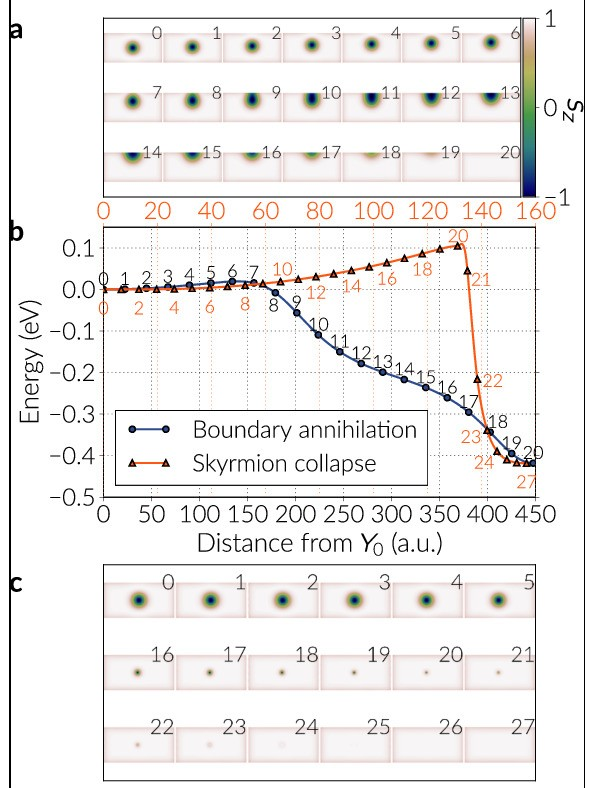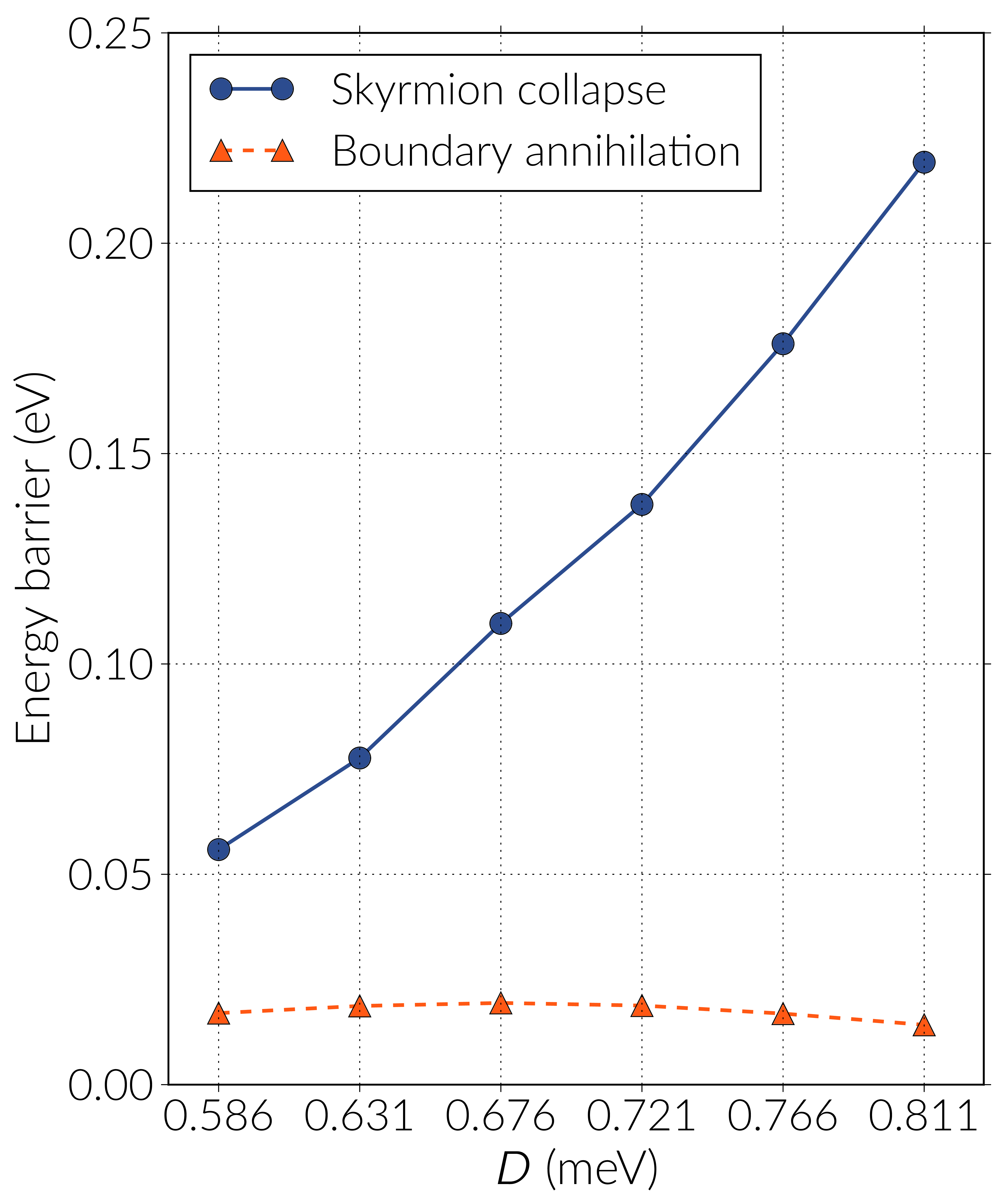# Thermal stability and topological protection of skyrmions in nanotracks

Summary of our recent publication about energy barriers associated to the destruction/nucleation mechanisms of skyrmions in ferromagnetic nanotracks with interfacial DMI:

Thermal stability and topological protection of skyrmions in nanotracks. David Cortés-Ortuño, Weiwei Wang, Marijan Beg, Ryan A. Pepper, Marc-Antonio Bisotti, Rebecca Carey, Mark Vousden, Thomas Kluyver, Ondrej Hovorka and Hans Fangohr

The paper focuses on the numerical calculation of transition paths of skyrmions stabilised in nanotracks with interfacial DMI. We use an atomistic model to describe the system and the Nudged Elastic Band Method to compute energy paths associated to the destruction/nucleation of an isolated skyrmion. These energy paths give us the magnitude of the energy barriers associated to different mechanisms of destroying/nucleating a skyrmion. We found out that the least energy transition is the one mediated by the boundary of the nanotrack.

## Nudged Elastic Band Method¶

The first Section of the paper introduces the Nudged Elastic Band Method, which is algorithm to compute minimum energy paths between two equilibrium states in magnetic systems. In the method, we fix the equilibrium states and create copies of the system along a specific path in the energy landscape, which can be any transition of the system that we use as initial state, creating a band of images or copies of the system. Consequently, we relax the band and the algorithm minimises the energy of every image, finding a path that cost the least energy. Images are kept together through a spring force (an elastic band) to avoid their clustering around the equilibrium states. The largest energy points in the final path will set an energy barrier separating the two equilibrium states.

More than one energy path can exist in the energy landscape and the one the algorithm finds will depend on the given initial state. The path with least energy will be the minimum enegy path and the most probable transition that the system will follow under any external excitation, such as thermal noise.We apply the NEBM to find minimum energy paths between the skyrmion and ferromagnetic (no-skyrmion) states, thus we set these two configurations as the fixed states int he energy band and use different initial paths.

## System¶

For the study of thermal stability of skyrmions in nanotracks we:

• Set an 40 nm wide, 80 nm long and 0.25 nm thick nanotrack, simulating a monolayer of magnetic material.
• Use Cobalt as the nanotrack material, which we assume is on top of a heavy metal to generate interfacial Dzyaloshinskii-Moriya interactions.
• Use a discrete spin model, hence we simulate the monolayer of atoms by setting a layer of 320x185 spins.
• Consider exchange, a strong out of plane uniaxial anisotropy, DMI and dipolar interactions.
• Vary the magnitude of the DMI in the range 0.586-0.811 meV in steps of 0.045 meV. In this range skyrmions of the Neel type are stabilised as metastable configurations and the global minimum is the ferromagnetic configuration. In this confined geometry the skyrmion does not require an external field for stabilisation and its size increases with larger DMI magnitudes. Dipolar interactions are important since they also influence the skyrmion size.

In Figure a below we show the skyrmion and ferromagnetic configuration energies where the scale has been shifted with respect to the energy of the ferromagnetic state with $D=0.405\,\text{meV}$. The diagrams in b illustrate these equilibrium states and the tilting of the spins at the sample edges.## Results¶

We found three destruction mechanisms for the skyrmion:

1. Destruction via the boundary of the nanotrack
2. Skyrmion collapse
3. Skyrmion destroyed by the emergence of a singularity

Path 3. has associated the largest energy barriers for every DMI magnitude. After extra relaxations with the NEBM (see the paper for details) this path usually relaxes to the skyrmion collapse path (2.), thus it is probably not very stable.

The path that costs the least energy is the one mediated by the boundary of the sample.

In the following Figure we see paths 1. and 2. for a skyrmion stabilised in a nanotrack with $D=0.676\,\text{meV}$. Snapshots in a are the images of Path 1. (the boundary mediated destruction) and snapshots in b show the images of the skyrmion collapse energy band. Energy magnitudes in c are shifted with respect to the skyrmion energy. In Figure c the energy barriers are given by the images with largest energy in the corresponding energy bands.Repeating these calculations for different DMI magnitudes we confirm that the annihilation of the skyrmion through the nanotrack edge has always the lowest energy barrier, which is a path that circumvents the topological protection of the skyrmion. The energy barrier for the skyrmion collapse increases almost linearly with larger DMI magnitudes.With these energy barrier magnitudes we can estimate the lifetime $\tau$ of the skyrmion using the Arrhenius-Neel law:

$$\tau = \tau_{0} \, \text{exp}\left(\frac{\Delta E}{k_{\text{B}} T} \right)$$

where $\Delta E$ is the energy barrier, $k_{\text{B}}$ is the Boltzmann constant, $T$ is the temperature and $\tau_{0}$ is related to the attempt frequency $\tau_{0}=f_{0}^{-1}$.

Assuming a value of the attempt frequency as 1 GHz, we can estimate the lifetime of a skyrmion in a nanotrack with $D=0.676\,\text{meV}$. For the boundary annihilation (barrier of approximately 0.0194 eV), which is the minimum energy path, we find a skyrmion lifetime of $\tau\approx 2.117\,\text{ns}$. For other DMI values the lifetime of the skyrmion is similar thus we notice a low stability of the skyrmion in this thin nanotrack system, in comparison with other technological applications such as perpendicular magnetic recording. Recent advances in skyrmion research such as skyrmions stabilised in multilayered systems or bulk DMI samples, or engineering the sample boundaries in a different fashion could potentially enhance the skyrmion lifetime and therefore its stability for a future skyrmion based magnetic recording device.

## Simulations¶

All the simulations of this study were done in Fidimag, where we implemented the Geodesic Nudged Elastic Band Method proposed by Bessarab et al. [Computer Physics Communications 196 (205)].

All the data and figures can be automatically generated through a Docker script in this repository: Data, where the scripts and data are carefully detailed.

We tested our NEBM implementation with the toy model example from Bessarab et al., which is analysed in the Supplementary material of the paper and which we published in the following repository: Example model.

Citations of the repositories are detailed in the paper (see below).

## Paper¶

The paper has been published in: Scientific Reports 7, Article number: 4060 (2017) with DOI doi:10.1038/s41598-017-03391-8, which can be found in this URL:

https://www.nature.com/articles/s41598-017-03391-8

### Bibtex entry¶

@Article{Cortes2017,
author   = {Cort{\'e}s-Ortu{\~n}o, David and Wang, Weiwei and Beg, Marijan and Pepper, Ryan A. and Bisotti, Marc-Antonio and Carey, Rebecca and Vousden, Mark and Kluyver, Thomas and Hovorka, Ondrej and Fangohr, Hans},
title    = {Thermal stability and topological protection of skyrmions in nanotracks},
journal  = {Scientific Reports},
year     = {2017},
volume   = {7},
number   = {1},
pages    = {4060},
issn     = {2045-2322},
abstract = {Magnetic skyrmions are hailed as a potential technology for data storage and other data processing devices. However, their stability against thermal fluctuations is an open question that must be answered before skyrmion-based devices can be designed. In this work, we study paths in the energy landscape via which the transition between the skyrmion and the uniform state can occur in interfacial Dzyaloshinskii-Moriya finite-sized systems. We find three mechanisms the system can take in the process of skyrmion nucleation or destruction and identify that the transition facilitated by the boundary has a significantly lower energy barrier than the other energy paths. This clearly demonstrates the lack of the skyrmion topological protection in finite-sized magnetic systems. Overall, the energy barriers of the system under investigation are too small for storage applications at room temperature, but research into device materials, geometry and design may be able to address this.},
doi      = {10.1038/s41598-017-03391-8},
url      = {http://dx.doi.org/10.1038/s41598-017-03391-8},
}